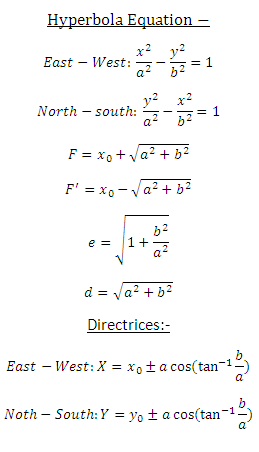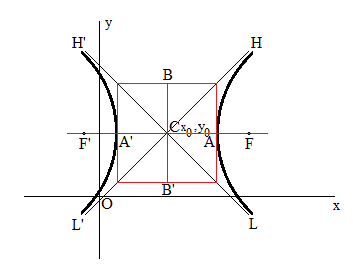# Hyperbola Calculator

This CalcTown calculator calculates the focii, eccentricity and directrices of a hyperbola.

#### Result

m
m
mWhere,

a = semi-major axis of the hyperbola.

b = semi-minor axis of the hyperbola.

x0 , y0 = center of the hyperbola.

F = 1st focus of the hyperbola.

F' = 2nd focus of the hyperbola.

e = eccentricity of the hyperbola.

d = distance from center to any one of the focii of the hyperbola.